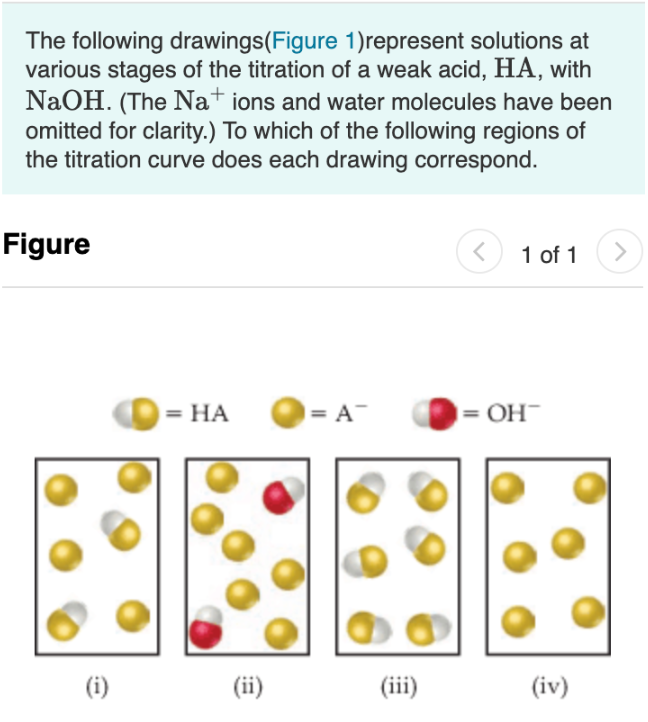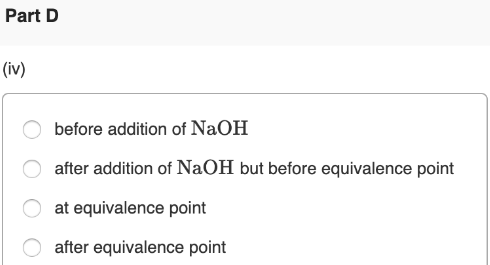# The following drawings (Figure 1) represent solutions at various stages of the titration os a weak acid., HA, with NaOH. (The Na^+ ions and water molecules have been omitted for clarity.) To which of the following regions of the titration curve does each drawing correspond. (iv) a) before addition of NaOH b) after addition of NaOH but before equivalence point c) at equivalence point d) after equivalence point